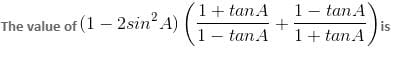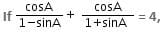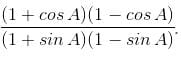CAT  >  Practice Questions Level 1: Trigonometry - 2

# Practice Questions Level 1: Trigonometry - 2 - Notes | Study Level-wise Practice Questions for CAT Preparation - CAT

 1 Crore+ students have signed up on EduRev. Have you?

This EduRev document offers 15 Multiple Choice Questions (MCQs) from the topic Trigonometry (Level - 1). These questions are of Level - 1 difficulty and will assist you in the preparation of CAT & other MBA exams. You can practice/attempt these CAT Multiple Choice Questions (MCQs) and check the explanations for a better understanding of the topic.

Question for Practice Questions Level 1: Trigonometry - 2
Try yourself:From a 60 m high building, the angles of depression of two cars on the opposite ends of the building are observed to be 60° and 30°. Find the distance between the cars, if the line joining them passes through the foot of the building.

Question for Practice Questions Level 1: Trigonometry - 2
Try yourself:If 10 sinθ = 6, then tanθ + cotθ =

Question for Practice Questions Level 1: Trigonometry - 2
Try yourself:A right triangle PQR is right-angled at Q. If tan R = 1/√3, then which of the following is the value of sin R?

Question for Practice Questions Level 1: Trigonometry - 2
Try yourself:If sin 30° = 1/2 , the value of expression tan 30° - sin 15° cos 15° is

Question for Practice Questions Level 1: Trigonometry - 2
Try yourself:In a right triangle ABC, right-angled at B, cot2 A = 9/16. What is the value of cos A?

Question for Practice Questions Level 1: Trigonometry - 2
Try yourself:Find the value of sec 30° cot 30° tan 30° cosec 30°.

Question for Practice Questions Level 1: Trigonometry - 2
Try yourself:If m = 1 + tan2θ and n = 1 + cot2θ, then m/n equals

Question for Practice Questions Level 1: Trigonometry - 2
Try yourself:If sinθ = 3/5, then find the value of (cosθ + tanθ + cotθ).

Question for Practice Questions Level 1: Trigonometry - 2
Try yourself:Question for Practice Questions Level 1: Trigonometry - 2
Try yourself:then what is the value of A?

Question for Practice Questions Level 1: Trigonometry - 2
Try yourself:From the top of a 10 m high building, the angle of elevation of the top of a tower is 45° and the angle of depression of its foot is 60°. How will you represent this situation diagrammatically?

Question for Practice Questions Level 1: Trigonometry - 2
Try yourself:The reduced form of cos6 x + sin6 x + 3 cos2 x.sin2 x is _______________.

Question for Practice Questions Level 1: Trigonometry - 2
Try yourself:tan2θ (1 + cot2θ) (cosec2θ + sec2θ)

Question for Practice Questions Level 1: Trigonometry - 2
Try yourself:If in a right triangle, tan A = 3/4 , then find the value ofQuestion for Practice Questions Level 1: Trigonometry - 2
Try yourself:From two points A and B on the opposite sides of a tower, the angles of elevation to the top of the tower are 45° and 30°, respectively. If the height of the tower is 120 m, then find the distance between A and B, corrected to two decimal places.

The document Practice Questions Level 1: Trigonometry - 2 - Notes | Study Level-wise Practice Questions for CAT Preparation - CAT is a part of the CAT Course Level-wise Practice Questions for CAT Preparation.
All you need of CAT at this link: CAT

## Level-wise Practice Questions for CAT Preparation

277 docs
 Use Code STAYHOME200 and get INR 200 additional OFF

## Level-wise Practice Questions for CAT Preparation

277 docs

### How to Prepare for CAT

Read our guide to prepare for CAT which is created by Toppers & the best Teachers

Track your progress, build streaks, highlight & save important lessons and more!

,

,

,

,

,

,

,

,

,

,

,

,

,

,

,

,

,

,

,

,

,

;